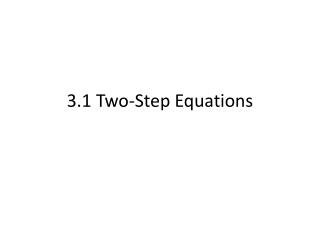DownloadDownload Presentation3.1 Two-Step Equations

# 3.1 Two-Step Equations

Télécharger la présentation## 3.1 Two-Step Equations

- - - - - - - - - - - - - - - - - - - - - - - - - - - E N D - - - - - - - - - - - - - - - - - - - - - - - - - - -
##### Presentation Transcript

1. 3.1 Two-Step Equations

2. An equation is like a seesaw. What does that mean?

3. Your goal is to isolate the variable.

4. In order to isolate the variable, you must undo whatever is being done. • Since you are undoing things, they must be done backwards.

5. DOING UNDOING P E MD AS AS MD E P Remember UNDOINGmeans solving equations

6. Doing things backwards means reverse the order of operations. 1. Undo any addition or subtraction. 2. Undo any multiplication or division. 3. Undo any exponents.